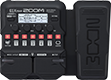# queen

Discussion in 'Zoom G1/G1X Four' started by Dygo Porto 13, Sep 22, 2021.

1. queenDevice: Zoom G1X Four
Firmware: 2.00

Optimized for: Phones/Speaker

Effects chain:('cvt_dev' patch):

Effect: "Comp" (Dynamics), active - "yes"
"Sense" = 6
"Attack" = Slow
"Tone" = 6
"Volume" = 80

Effect: "VioletDST" (Overdrive / Distortion), active - "yes"
"Gain" = 80
"Tone" = 40
"Voice" = 2
"Volume" = 75

Effect: "Gt GEQ" (Filter), active - "yes"
"160" = 5.0
"400" = 2.0
"800" = 2.0
"3.2k" = 0.0
"6.4k" = -4.0
"12k" = -1.5
"VOL" = 80

Effect: "Step" (Filter), active - "yes"
"Depth" = 90
"Rate" = 30
"Resonance" = 8
"Shape" = 10

Effect: "Delay" (Delay), active - "yes"
"Time" = 400
"F.B." = 20
"Mix" = 30
"Tail" = Off

Patch Volume: 100